Table of ContentOpen Access

ARTICLE

# The Coupled Thermo-Chemo-Mechanical Peridynamics for ZrB2 Ceramics Ablation Behavior

Yuanzhe Li1, Qiwen Liu2,*, Lisheng Liu2, Hai Mei2
1 Department of Engineering Structure and Mechanics, Wuhan University of Technology, Wuhan, 430070, China
2 Hubei Key Laboratory of Theory and Application of Advanced Materials Mechanics, Wuhan University of Technology, Wuhan, 430070, China
* Corresponding Author: Qiwen Liu. Email:(This article belongs to this Special Issue: Peridynamics and its Current Progress)

Computer Modeling in Engineering & Sciences 2023, 135(1), 417-439. https://doi.org/10.32604/cmes.2022.021258

Received 05 January 2022; Accepted 19 May 2022; Issue published 29 September 2022

### Abstract

The ablation of ultra-high-temperature ceramics (UTHCs) is a complex physicochemical process including mechanical behavior, temperature effect, and chemical reactions. In order to realize the structural optimization and functional design of ultra-high temperature ceramics, a coupled thermo-chemo-mechanical bond-based peridynamics (PD) model is proposed based on the ZrB2 ceramics oxidation kinetics model and coupled thermo-mechanical bond-based peridynamics. Compared with the traditional coupled thermo-mechanical model, the proposed model considers the influence of chemical reaction process on the ablation resistance of ceramic materials. In order to verify the reliability of the proposed model, the thermo-mechanical coupling model, damage model and oxidation kinetic model are established respectively to investigate the applicability of the proposed model proposed in dealing with thermo-mechanical coupling, crack propagation, and chemical reaction, and the results show that the model is reliable. Finally, the coupled thermo-mechanical model and coupled thermo-chemo-mechanical model are used to simulate the crack propagation process of the plate under the thermal shock load, and the results show that the oxide layer plays a good role in preventing heat transfer and protecting the internal materials. Based on the PD fully coupled thermo-mechanical model, this paper innovatively introduces the oxidation kinetic model to analyze the influence of parameter changes caused by oxide layer growth and chemical growth strain on the thermal protection ability of ceramics. The proposed model provides an effective simulation technology for the structural design of UTHCs.

### Keywords

ZrB2 ceramics; ablation; coupled thermo-chemo-mechanic; peridynamics model; oxide layer

1  Introduction

Ultra-high-temperature ceramics (UHTCs) refer to a class of ceramic materials that can maintain physical and chemical stability under high-temperature and oxygen atmospheres. They are mainly multi-component composite ceramics composed of borides, carbides, nitrides and other transition metal compounds based on hafnium, zirconium, and molybdenum, such as ZrB2, HfB2, TaC, HfC, ZrC, and HfN . UHTCs have the characteristics of ultra-high temperature resistance, high thermal conductivity, and high strength. They can be used as heat-bearing structural components such as the nose cone and wing leading edge of reusable spacecraft. In order to achieve the goal of lightweight and high performance of UHTCs, the optimal design of UHTCs ceramic materials must be realized based on the understanding of UHTCs ablation mechanism and ablation resistance.

The research on UHTCs began as early as 1960s , but it was difficult to obtain excellent performance due to the technical constraints at that time. In the late 1990s, the urgent demand for ultra-high-temperature non-ablative thermal protective materials has brought them under the limelight . Among currently studied UHTCs, ZrB2-and HfB2-based ceramics render good anti-oxidation and ablation properties, and achieve long-term stability under ultra-high temperature, showing promise as non-ablative ultra-high-temperature heat protection materials. In 2007, Han et al.  have investigated the oxidation resistance of ZrB2-SiC composite under the oxyacetylene flame. At greater than 2200°C, the mass loss rate and linear oxidation rate of the composite after 10 min were found to be 0.23 mg/s and 0.66 μm/s, respectively. Also, a dense ZrO2 layer was formed on the oxidized surface, and no obvious cracks and denudation were observed. Then, in 2011, Wang et al.  have studied the ablation behavior of C/C-ZrC composites under the oxyacetylene flame and demonstrated that the oxidation reaction generated a dense ZrO2 layer when ZrC was exposed to a high-temperature oxygen environment. When the temperature is increased to 2800°C, the solid ZrO2 transforms into a molten state and covers the material surface. The formation of a dense ZrO2 layer prevents further ablation of C/C-ZrC composites. In 2020, Qing et al.  have studied the ablation mechanism of C/C-ZrC-SiC composites containing ZrB2-SiC multiphase ceramic rods. The results demonstrated that the ablation mechanism of Zr-Si-B-C multiphase ceramics in the temperature range of 25°C to 3000°C generates B2O3 film in the low-temperature zone, SiO2 film in the medium-temperature zone and ZrO2 film in the high-temperature zone. This Zr-Si-B-C multiphase ceramic ensures that the material will not be seriously ablated in the low-and high-temperature regimes. Based on these studies, it can be found that a liquid or solid oxide layer is formed on the surface of ablative UHTCs under a high-temperature aerobic environment, which can effectively inhibit or prevent the further reaction between external oxygen and ceramic matrix to reduce the amount of ablation.

At present, the ablation resistance mechanism of ZrB2 ceramic materials has been clearly understood, but the numerical theoretical research on ablation has not been fully studied. Considering the complexity of the actual service environment, a numerical model needs to be established to describe various complex physical and chemical reactions during the ablation process of UHTCs, and this numerical model can be used to study the factors affecting the ablation process, as well as the influence behavior and mathematical quantitative function of each factor against ablation, so as to predict the ablation rate, temperature distribution, structural deformation and denudation damage. These works are helpful to the structural optimization and functional design of UHTCs. In 2005, Fahrenholtz  has proposed a theoretical model that can calculate the oxygen partial pressure in the coexistence of ZrB2, ZrO2 and B2O3. The proposed model can also predict the mass fraction of liquid B2O3 remaining in porous solid ZrO2. The model was experimentally verified by Talmy et al. . Then, Fahrenholtz  has analyzed the oxidation behavior of ZrB2-SiC UTHCs and proposed the reaction sequence, oxide composition and formation rate during the oxidation process. Based on these studies, Parthasarathy et al. [10,11] have proposed an oxidation kinetic model that can describe oxidation process of diboride UHTCs, such as ZrB2, HfB2 and TiB2. Obviously, the ultra-high-temperature ablation of ceramics needs to comprehensively consider the interaction of temperature field, structural deformation and chemical reactions. Zhou et al.  have extended the oxidation kinetics model of ZrB2 and proposed a thermal force coupling model, which could describe the multi-field coupling behavior of ZrB2 during high-temperature oxidation and predict the stress state of ceramic matrix and oxide layer during ablation process. Similarly, Wang et al.  have proposed a chemical force coupling model based on Parthasarathy’s oxidation kinetics model, comparing and analyzing the effect of different volume fractions of SiC on ablation resistance of ZrB2-SiC ceramics.

Though these studies can describe the changes in chemical reactions during the ablation process of UHTCs, the ultra-high-temperature ablation of ceramics is also accompanied by some discontinuous problems, such as thermal response, material damage, boundary movement and structural failure. Obviously, the previous studies did not consider these discontinuities. However, the peridynamics (PD) render unique advantages in dealing with discontinuities. The peridynamic theory was first proposed by Silling as a nonlocal theory , considering the advantages of meshless method and molecular dynamics, and avoiding the limitations of molecular dynamics on a computational scale. At the same time, material damage is a part of the PD constitutive relationship. So, the damage can develop spontaneously in the PD model . With the continuous development of PD theory research, the coupled thermo-mechanical theory based on PD also appears. In 2014, Oterkus et al.  have deduced the fully-coupled peridynamic thermomechanics and given the bond-based PD equation and its dimensionless form. Subsequently, Liao et al.  have applied PD to the numerical simulations of transient heat transfer and thermo-mechanical coupling of functionally-graded materials. Wang et al. [18,19] proposed a weak coupled thermo-mechanical ordinary state-based peridynamic model to investigate the thermal cracking behavior of rocks under borehole heating and the crack propagation of ceramic plates in water quenching experiments respectively. The PD’s analysis results are consistent with the experimental observations. Chen et al.  have proposed a refined thermo-mechanical fully coupled peridynamics. This method did not require micro-conductivity and could directly represent the temperature deformation term by the integral of displacement difference. Based on the proposed model, a homogeneous concrete cylinder under thermal load was simulated from two-and three-dimensional angles, where the crack propagation results exhibited a good agreement with the experimental data. Crack propagation plays an important role in the failure analysis of brittle materials , and the crack initiation of ultra-high temperature ceramic materials is inevitable under extreme thermal load. Therefore, it is very important to characterize the crack initiation and development in numerical simulation. The bond-based PD method has been proved to be reliable in dealing with crack initiation and development . Wang et al. [23,24] proposed an improved bond-based peridynamic coupled thermo-mechanical model based on multi-rate time integration. The model includes crack initiation and development, which can accurately simulate the crack initiation and development of brittle solids under thermal shock load, and accurately predict the radial and circumferential thermal crack growth of fuel rods. In recent years, there are many studies on the fracture mode of PD. Yu et al.  re-examined the relation between the critical bond stretch and the energy release rate for different crack types in Peridynamics by using Griffith’s “surface energy approach” and revealed that the choice of the critical bond stretch will be crucial for fracture model. Based on coupled thermo-mechanical peridynamic theory, Song et al.  established a coupled thermal mechanical periodic model with modified failure criterion suitable for de-icing technology. Li et al.  proposed an improved peridynamic stress expression, which can well predict the stress state at the crack tip.

The thermo-chemo-mechanical coupling needs to comprehensively consider the complex interactions between temperature field, chemical reaction and structural displacement. In recent years, based on the general thermodynamic principle and combined with the experimental data, scholars have studied the thermal force coupling of continuous solid materials [28,29]. Although these coupled thermo-chemo-mechanical models have rendered some good results in their respective research fields, these numerical theories are not suitable for dealing with discontinuities, such as damage and cracking. The ablation of UTHCs is a typical nonlinear and discontinuous problem. The PD theory can better deal with the ablation problem. PD solve various mechanical or thermal problems by using the integral equations based on non-local interaction to replace the differential equations of traditional continuum mechanics.

Based on single-phase ZrB2 ceramic, a novel thermo-chemo-mechanical coupling equation is established by combining the bond-based peridynamics (BB-PD) coupled thermo-mechanical with the high-temperature oxidation kinetics theory of single-phase ZrB2 ceramics in this paper. In Section 2, we will introduce the ablation mechanism, high-temperature oxidation kinetics theory of single-phase ZrB2 ceramics, and the bond-based peridynamic thermo-chemo-mechanical coupling model. Then, we present the initial boundary conditions of the model and damage criteria. Based on the coupled thermo-chemo-mechanical model (Section 2), the numerical simulation method is presented in Section 3. Furthermore, Section 4 shows that the proposed BB-PD coupled thermo-chemo-mechanical model is verified by numerical simulations and examples. Based on ZrB2 ablation, the calculation results of the fully coupled thermo-mechanical model and the coupled thermo-chemo-mechanical model are compared and analyzed. Finally, Section 5 presents the key conclusions of the current work.

2  The Coupled Thermo-Chemo-Mechanical Peridynamic Model

2.1 ZrB2 Ceramic Ablation Mechanism and Oxidation Kinetics Model

2.1.1 Ablation Mechanism of Single-Phase ZrB2 Ceramics

The relevant experimental results  reveal that, with the change of surface ablation temperature of ZrB2 ceramics, the following chemical reactions occur during the ablation process:

ZrB2(cr)+(5/2)O2(g)ZrO2(cr)+B2O3(l)T>873K(1)

B2O3(l)B2O3(g)T>1273K(2)

ZrB2(cr)+(5/2)O2(g)ZrO2(cr)+B2O3(g)T>2073K(3)

The abovementioned reaction process is described in Fig. 1. When the temperature exceeds 800 K, the oxidation of ZrB2 in the oxygen environment begins to become obvious and ZrB2 oxidizes to produce solid particles of ZrO2 and liquid B2O3. The former serves as a skeleton and the latter serves as a filling and cover. One should note that the oxygen needs to diffuse through the oxide film composed of ZrO2 and B2O3 in order to react with the underlying matrix material. The oxygen transport rate in the liquid film is very low, which drastically decreases the ablation rate. This is a typical inert oxidation phenomenon. The purpose of protecting the material is achieved by the slight oxidation of the material. When the temperature is higher than 1273 K, the evaporation of B2O3 on the surface becomes significant and, with the increase of temperature, B2O3 gradually shrinks into the pores of ZrO2. When the temperature is higher than 2073 K, the volatilization rate of B2O3 becomes greater than the generation rate and almost only porous ZrO2 remains in the oxide layer.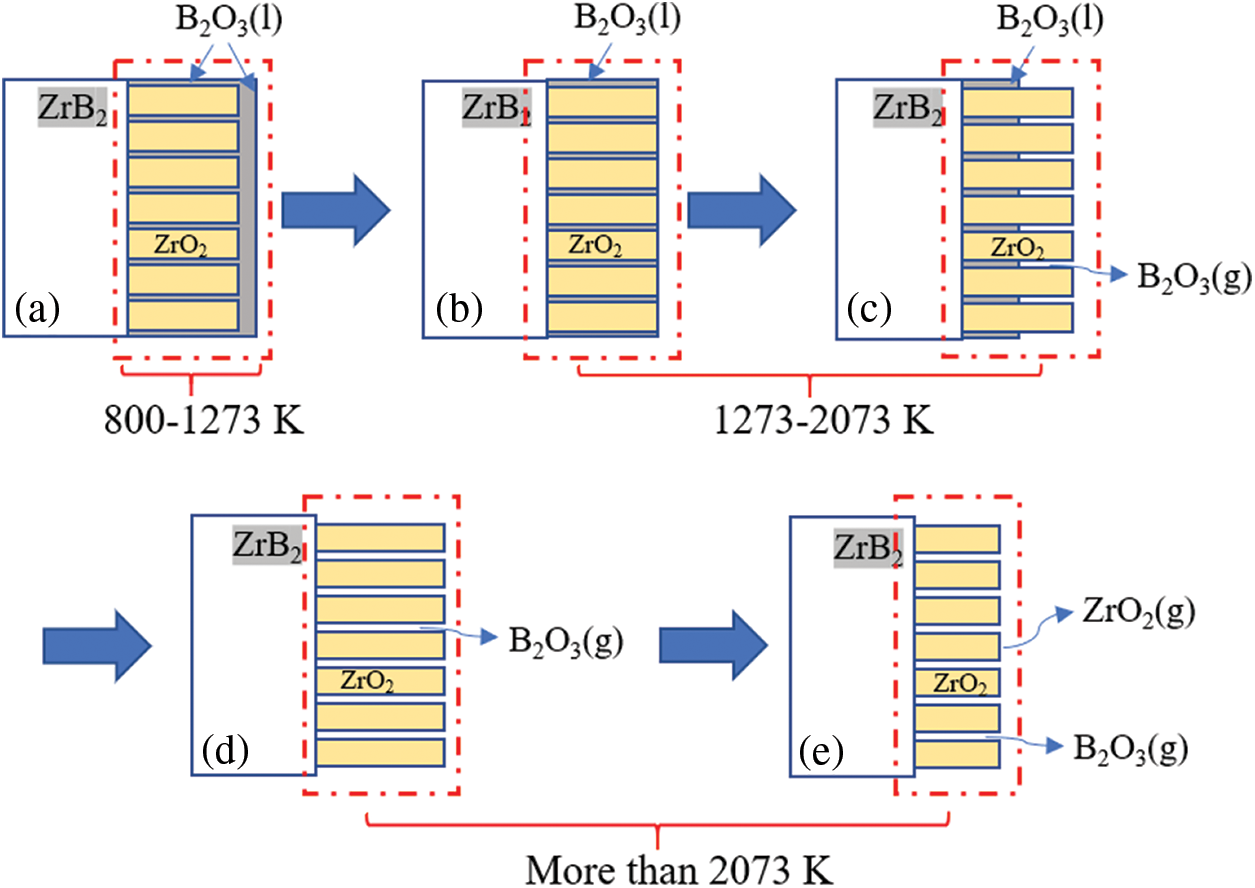Figure 1: Schematic diagram of ZrB2 oxidation process with respect to temperature

2.1.2 ZrB2 Ceramic Oxidation Kinetics Model

In this section, based on the oxidation process (Fig. 1), the oxidation kinetics model of single-phase ZrB2 ceramics is established (Fig. 2). This model can represent the ablation mechanism of ZrB2 in the intermediate temperature range (1273–2073 K) in a high-temperature aerobic environment.Figure 2: Schematic diagram of the oxidation model of ZrB2

As shown in Fig. 2, reaction (1) occurs at the matrix-oxidation interface (interface-1) and reaction (2) occurs at interface-2. The evolution of oxide layer thickness during oxidation can be calculated by the following equation :

L2=2[25(MZrO2ρZrO2)(f1f)DO2CO23CO22(1q)]t(4)

where ρZrO2 refers to ZrO2 density, kg/m3; MZrO2 refers to ZrO2 molar mass, kg/mol; f refers to the porosity of porous ZrO2 oxide layer; DO2 denotes the effective diffusion coefficient of oxygen; and CO22 and CO23 refer to the O2 concentration at 2 and 3 reaction interfaces, respectively. CO23 can be the oxygen concentration in the atmosphere, which is a constant, while CO22 can be calculated by the following expression :

CO22=CO2352DB2O3DO2(CB2O32CB2O33)(5)

Similarly, DB2O3 represents the effective diffusion coefficient of B2O3, CB22O3 and CB2O33 represents the B2O3 concentration at the 2 and 3 reaction interfaces, respectively. The B2O3 concentration in the environment can be taken as 0, like CB23O3=0, while the B2O3 concentration at the 2-2 interface can be calculated by the following equation :

CB2O32=3×108exp(45686T)/RT(6)

where R is the gas constant (8.314 J/(mol·K)). For q in Eq. (4), it is a constant that varies with temperature, and related to the liquid B2O3 layer thickness (hint) and the oxide layer thickness (L), which can be defined as:

hint=qL(7)

For the calculation of hint, DO2 and DB2O3 please refer to Parthasarathy et al. , which will not be described in detail here. The growth strain appears with the growth of oxide layer. Based on the phenomenon of oxide layer growth, Clarke  has proposed the following equation to describe the growth strain and oxide layer thickness growth rate, which be written as:

ε˙g(t)=Doxh˙ox(t)(8)

where εg represents the chemical growth strain of the oxide layer; Dox denotes the chemical reaction growth coefficient; hox(t) corresponds to the thickness of the oxide layer, which is L in Eq. (4).

2.2 Bond-Based Peridynamic Equation of Motion

The peridynamic theory was first proposed by Silling et al.  in 2000, which is commonly referred as the bond-based peridynamics (BB-PD) theory. In BB-PD, the motion equation of mechanics can be expressed as:

ρ(x)u¨(x,t)=Hxf(uu,xx,t)dVx^+b(x,t)(9)

where ρ(x) represents the mass density of the material point x. u¨(x,t) represents the second derivative of the displacement u(x,t) of the material point x at time t. f(uu,xx,t) is the force response function, which is defined as the force loss per unit volume square applied by the material point x on the material point x, and b(x,t) represents the physical density of the material point x at time t. As shown in Fig. 3, y and y refer to the position vectors of the material points x and x after displacements u and u, respectively.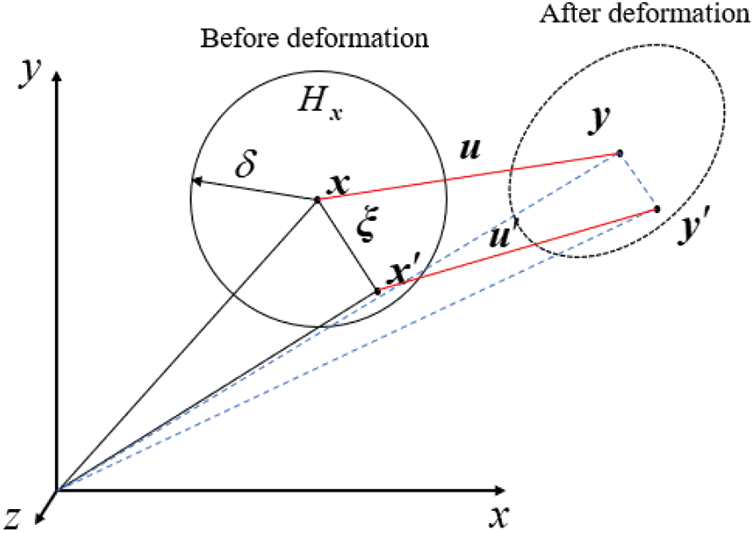Figure 3: The interactions of material points x and x′ before and after deformation

In the bond-based peridynamic theory, the force response function is the force density vector. Considering the effect of temperature change on deformation, the force density vector can be expressed as:

f(uu,xx,t)=c[s(uu,xx,t)αT(uu,xx,t)]yy|yy|(10)

where T(uu,xx,t) represents the average value of the temperature change of material point x and

s(uu,xx,t)=|yy||xx||yy|(11)

Combined with Eq. (10), s(uu,xx,t) represents the distance between two material points and αT(uu,xx,t) refers to the thermal expansion deformation caused by temperature change. In classical continuum mechanics, the elastic strain can be expressed as:

εe=εtotεT(12)

Herein, εe represents elastic strain. εtot refers to total strain and εT corresponds to thermal strain. It can be found that εtotεT corresponds to sαTavg in Eq. (10). Combined with the oxidation kinetics in Section 2.1, the total strain of the oxide layer can be expressed as:

εtot=εe+εT+εg(13)

Eq. (13) can be re-written as εe=εtotεTεg to replace sαTavg of Eq. (10), which can be equivalent that the elongation of the bond minus the thermal expansion deformation and the chemical reaction deformation. At this time, the bond force response function can be written as:

f(uu,xx,t)=c(sαTavgDoxhox)yy|yy|(14)

Combined with Eqs. (9) and (13), the BB-PD motion equation considering temperature effect and chemical growth strain can be written as:

ρu¨(x,t)=Hξ+η|ξ+η|c(sαTavgdoxhox)dV+b(x,t)(15)

where c refers to the peridynamic parameter and the micro-elastic modulus. In the two-dimensional plane stress problem, the micro elastic modulus of isotropic material can be given as: c=9E/(πhδ3) where E represents the elastic modulus. The definitions of initial relative position vector and relative displacement vector are ξ=xx and η=uu, respectively. α represents the coefficient of linear thermal expansion and Tavg refers to the average temperature change between material points x and x, which can be defined as:

Tavg=(T+T)/2(16)

2.3 Bond-Based Peridynamic Equation of Heat Conduction

Based on Fourier’s law of heat transfer, the bond-based PD thermal conduction equation can be expressed as:

ρ(x)cvT˙(x,t)=Hfh(T˙,T,x,x,t)dVx+hs(x,t)(17)

where cv refers to the specific heat capacity of the substance, T(x,t) represents the temperature of the material point x at time t, hs(x,t) represents the heat source density, and fh(T˙,T,x,x,t) corresponds to the thermal response function, which is solely governed by the interactions between material points x and x and can be expressed as:

fh(T˙,T,x,x,t)=κT(x,t)T(x,t)|xx|(18)

Introducing the temperature change term c2αe˙ due to deformation and substituting Eq. (18) into Eq. (17), the bond-based PD thermal conduction equation containing the deformation effect term on temperature can be written as:

ρcVT˙(x,t)=H(κT(x,t)T(x,t)|xx|T0c2αe˙)dV+hs(x,t)(19)

where e˙ represents the time derivative of the amount of elongation between material points and can be defined as:

e=|ξ+η||ξ|(20)

its time derivative can be written as:

e˙=ξ+η|ξ+η|η˙(21)

where κ represents the micro-thermal conductivity of PD, which is related to the thermal conductivity (kT) of the classical thermodynamic theory and micro-thermal conductivity is κ=6kT/(πhδ3) in two-dimensional problems.

In summary, Eqs. (4), (15) and (19) form the bond-based peridynamic thermo-chemo-mechanical coupling equation for ultra-high temperature ablation of ZrB2 ceramics. The proposed model is a simple thermo-chemo-mechanical coupling. The chemical reaction only affects the deformation of the oxide layer, while the effect of the chemical reaction is not considered for the unreacted matrix. However, it should be noted that the model can perform fully coupled thermoelastic analysis. In this model, the structural deformation and temperature field affect each other. The detailed numerical realization method is described in Section 3.

2.4 Initial and Boundary Conditions

2.4.1 Thermal Boundary Conditions

In bond-based peridynamic thermal diffusion theory, an initial temperature is set for all material points. If the boundary temperature distribution TBC(x,t) is known, the boundary temperature can be applied directly to the material points of the boundary layer RBC, which can be given as:

T(x,t)=TBC(x,t),xRBC(22)

For the heat flow boundary, it is necessary to convert the heat flow into the heat source density and apply the heat source density to the material points of the boundary. Assuming that the heat flux at the boundary RBC is qBC, the heat flux at the boundary RBC can be given as:

hs(x,t)=qBCnΔ,xRBC(23)

If there is a temperature difference between the material surface (RBC) and surrounding environment, the temperature difference will cause the heat transfer between the material and environment medium. Hence, the temperature boundary belongs to the heat convection boundary, which can be given as:

hs(x,t)=h(TT(x,t))Δ,xRBC(24)

where h refers to the convection heat transfer coefficient between boundary (RBC) and surrounding environment. Δ is the thickness of the boundary RBC.

2.4.2 Mechanical Boundary Conditions

The boundary conditions in dynamics’ problem usually include displacement boundary, velocity boundary, acceleration boundary and force boundary. In peridynamic theory, displacement constraints, velocity constraints and acceleration constraints are represented by vector UBC, vector VBC and vector aBC, respectively. These constraints can be applied directly to the particles of the outer boundary (Rs):

u(x,t)=UBC,v(x,t)=VBC,a(x,t)=aBC,xRs(25)

When distributed pressure p(x,t) or concentrated force P(t) are applied on the boundary (RBC) layer surface, the body force density vectors can be expressed as:

b(x,t)=pBCΔn,xRBC,b(x,t)=PBCVx,xRBC(26)

2.4.3 Chemical Initial Conditions

It is assumed that the high-temperature oxidation of ZrB2 only takes place at the interface under the thermal load and only the initial conditions of chemical reaction need to be considered. In the oxidation kinetics model of Section 2.1, only the initial concentration of oxygen CO23 in the chemical reaction needs to be considered. The initial concentration of oxygen CO23 remains constant throughout the chemical reaction.

2.5 Damage Model

In the solution of peridynamics, the displacement of each material point and elongation between each pair of material points are calculated. A time scalar function μ(x,x,t)  is introduced to deal with the damage caused by temperature changes. The criteria for this time scalar function can be given as:

μ (x,x,t)={ 1 sαTavgDoxhox(t)<s00 sαTavgDoxhox(t)s0(27)

where s0 refers to the critical elongation of the bond in the planar stress state and can be expressed as:

s0=4πG09Eδ(28)

where the material’s critical energy release rate G0 is related to the fracture toughness KIC, and their relationship with the elastic modulus E can be expressed as: G0=KIC2/E. When μij=1, it indicates that the bond between material points x and x is intact. When μij=0, it indicates that the bond between material points x and x is broken. Herein, the temperature bond is not adherent to the force bond and, when the force bond is broken, the heat transfer efficiency of the temperature bond is weakened. The corresponding numerical expression can be given as:

μijthemal(t)={ 1 sij αijTavgDoxhox(t)<s00.6 sijαijTavgDoxhox(t)s0and0φi(t)<0.20.3 sijαijTavgDoxhox(t)s0and0.2φi(t)<0.40.01 sijαijTavgDoxhox(t)s0and0.4φi(t)<1(29)

where φi(t) represents the local damage to the material point xi, which is related to the time-dependent function μij of the bond and can be defined as:

φi(t)=1Hxμij(t)dVjHxdVj(30)

When φ=1, it indicates that all bonds within the neighborhood of the substance points are broken. When φ=0, it indicates that the bonds within the neighborhood of the material points are intact.

3  Numerical Implementation

3.1 Numerical Discretization

Combined with the Eqs. (15) and (19), the discrete form of the two-dimensional bond-based peridynamic coupled thermo-chemo-mechanical equation considering damage can be given as:

ρiciT˙i(t)=j=1Niμijthemal(κijTjTi|ξij|T0cijαij2e˙ij)Vj+hsi(t)(31)

ρiu¨i(t)=j=1Niμijcij(sijαijTavgDoxhox)ξij+ηij|ξij+ηij|Vj+bi(t)(32)

where the neighborhood volume of each material point xi is Vi and the neighborhood contains Ni interacting material point xj.

Eq. (32) shows that hox needs to be solved for solving this coupled formula further, while the thickness of the oxide layer at t(n+1) can be obtained from the calculations, as given below:

hox(tn+1)=hox(tn)+Δhox(tn)(33)

The incremental form of the oxide layer thickness calculation using Eq. (4) can be given as:

Δhox(tn)=Δt[15(MZrO2ρZrO2)(f1f)DO2CO23CO22(1q)tn]1/2(34)

It is important to note that the solution of the oxidation kinetics model belongs to a semi-independent process, which requires the distribution of temperature field. The bond-based PD coupled thermo-chemo-mechanical equation in this paper is based on the equations of motion and thermal conduction, and the kinetic equation of oxidation is used as an auxiliary. The calculation results of Eq. (33) need to be substituted into Eq. (32) to achieve the coupled solution of thermal conduction (Eq. (31)) and motion (Eq. (32)).

In addition, the material parameters of the newly generated oxide layer during the ablation process are quite different from the original substrate material. As shown in Fig. 4, we ignore the material loss and the oxide layer replaces the base material in the original position from the outside to the inside, and updates the material parameters of the oxide layer at each time step.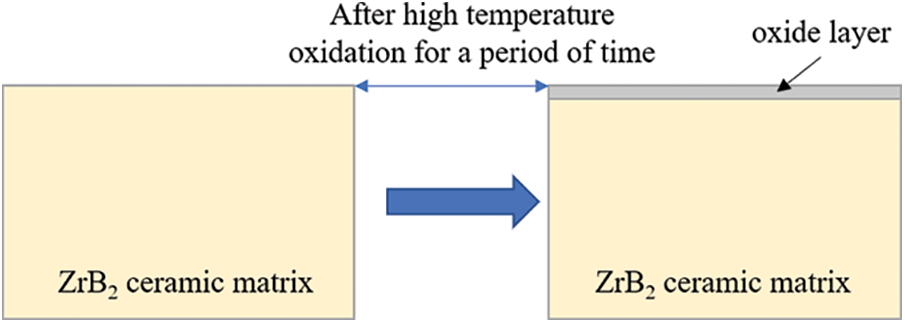Figure 4: The evolution of oxide layer during ablation

3.2 Solution Procedue

For the numerical solution of a classical fully-coupled thermo-mechanical equation, there are two generic methods, i.e., a single-step algorithm and an interleaved algorithm. In the single-step algorithm, also called the ensemble algorithm, time steps are simultaneously applied to the whole system of equations and multiple unknown variables are solved simultaneously. If the time integral of a single-step algorithm is implicit, absolute stability can usually be achieved, but it leads to a massive solution system. The interleaved algorithm partitions the coupled equations, solving the temperature and displacement fields with different time display algorithms. The interleaved algorithm can achieve a stable solution only under certain conditions.

Herein, according to the characteristics of the discrete form of the bond-based peridynamic equation and considering the particularity of the coupled thermo-chemo-mechanical model, an interleaved algorithm is used for the solution of coupled thermo-mechanical equations. The system is automatically divided according to the displacement field and temperature field, where the equation of motion is used for solving the displacement field and the equation of heat conduction is used for solving the temperature field. The solution of both equations is calculated by explicit time integration. Also, the computational equation of oxidation kinetics is relatively simple and can be solved directly.

The numerical calculations of the coupled thermo-chemo-mechanical model during the ablation of ultrahigh-temperature ceramics based on BB-PD mainly includes the following steps:

1.    Defining the array, initializing variables and generating the physical numerical model;

2.    Searching each material point within the neighborhood particles;

3.    Initializing time-dependent function and surface correction factor;

4.    Starting the first-time step cycle and calculating the temperature field;

5.    Starting oxidation kinetics calculation and calculating the oxide layer film thickness produced due to the chemical reaction;

6.    Starting the calculations of displacement field and reassigning material parameters to the material points of the oxide layer;

7.    Judging whether the calculations are terminated and, if no, proceeding to Step 4 to start the next time step cycle;

8.    Ending the cycle and obtaining the output results at each time step.

Fig. 5 shows the numerical calculations flow chart for solving the coupled thermo-chemo-mechanical model.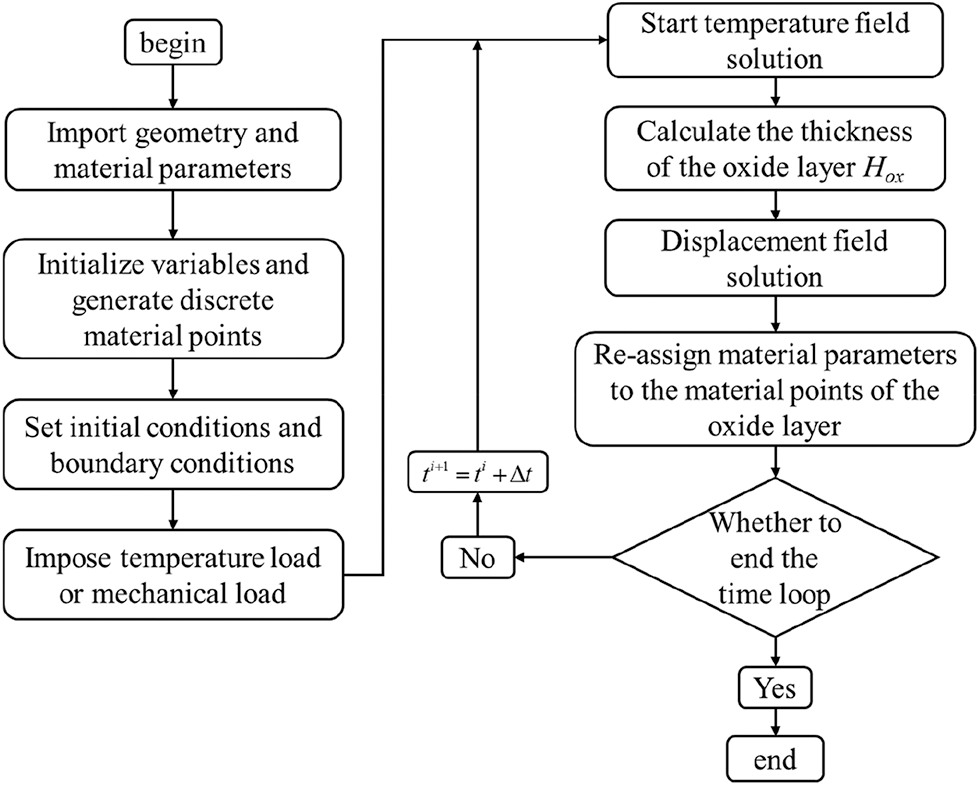Figure 5: The flow diagram of numerical simulations

3.3 Numerical Stability Condition

The time integral of PD heat conduction equation is calculated by forward difference method, which is conditionally stable. In order to prevent the divergence of numerical solutions, it is necessary to give a stability condition to limit the time step of thermal diffusion. Referring to the existing literature, similar to the method used by Silling et al. , in order to meet the stability conditions of thermal diffusion, the critical time step ΔtcrTH of temperature field calculation can be calculated by the following formula:

ΔtcrTH=ρ(x)(i)cV(i)j=1Nκ|ξ(i)(j)|V(j)(35)

where, ΔtcrTH is the critical thermal diffusion time step. In order to meet the stability conditions of numerical calculation of thermal diffusion, the thermal diffusion time step ΔtTH should meet the following conditions:

ΔtTH<ΔtcrTH(36)

ΔtTH is the time step of thermal diffusion calculation. After each thermal diffusion time step, the Adaptive Dynamic Relaxation (ADR) method  is used by introducing artificial damping to the solution system, and the static or quasi-static solution in the dynamic problem is solved by the explicit forward difference time integration method. The static or quasi-static solution is the steady-state part of the instantaneous response solution. When the static or quasi-static solution is obtained, the displacement and damage caused by the coupled thermo-mechanical material points will be solved as the initial parameters of the next thermal diffusion time step. In the ADR method, the time step of displacement calculation is ΔtcnME=1s.

4  Numerical Examples and Discussion

In this section, first, the correctness and accuracy of the bond-based peridynamic coupled thermo-chemo-mechanical numerical model are verified through three benchmark numerical examples: the fully coupled thermo-mechanical analysis of two-dimensional flat plate under temperature load; crack propagation simulations of the ceramic plate under cold shock, and evolution of oxide layer thickness of ZrB2 ceramic under high-temperature environments. In the fully coupled thermo-mechanical analysis of a two-dimensional flat plate under temperature load, the influence of neighborhood size and particle size on PD calculation results is investigated by comparing the calculation results under different neighborhood sizes and particle sizes with the analytical solution and the results of ABAQUS. The chemical reaction and coupled thermo-mechanical parts are verified separately because there is no corresponding ablation experimental research. Hence, the calculation results of the ZrB2 oxidation kinetics model can only be compared with the relevant high-temperature oxidation experiments of ZrB2. Accordingly, the coupled thermo-mechanical part can be verified by commercial software or an analytical method. Finally, considering the growth and evolution of damage and oxide layer, the coupled thermo-chemo-mechanical model and fully-coupled thermo-mechanical model are compared and analyzed.

4.1 Verification of the Fully Coupled Thermo-Mechanical and Convergence Study

As shown in Fig. 6, the side length of the square plate is 1 m, the initial temperature of the plate is 0°C, and the top side is experiencing a thermal load of 1°C. Then, the constraints in the x-direction are restricted at the left and right boundaries of the plate and the constraints in the y-direction are restricted at the lower boundary. Table 1 presents the parameters of the homogeneous plate. The Poisson’s ratio is 0.333. Points a and b are located on the vertical central axis of the plate, respectively, at the top and the middle, as shown in Fig. 6.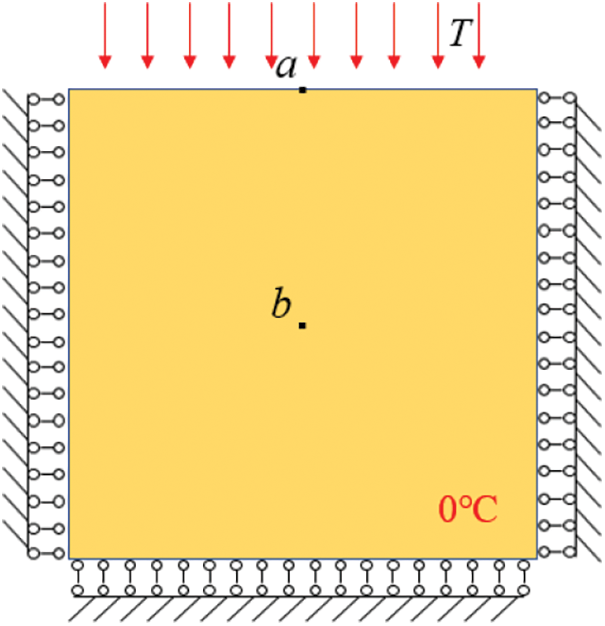Figure 6: The top boundary of the plate is under thermal load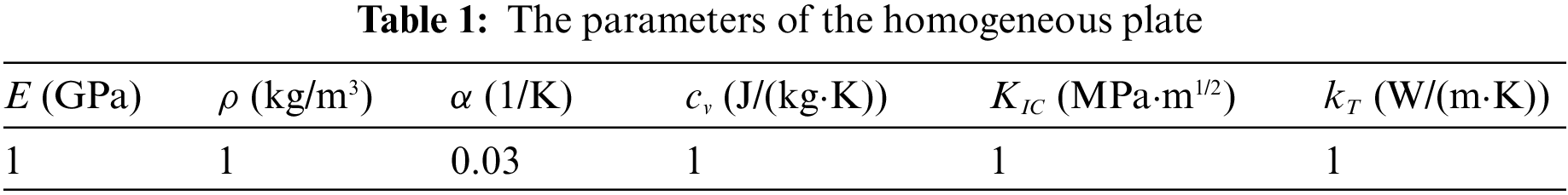4.1.1 Influence of Neighborhood Size

In peridynamic theory, the material point only interacts with other material points in the neighborhood of this point, so the size of horizon will affect the numerical calculation results of PD. In order to investigate the influence of horizon size on numerical calculation, this section fixes the distance between material points as Δx=0.005m and horizon size is set as δ=mΔx. The time step is Δt=0.00001s. The plate model is divided into 200×200=40000 particles, select different horizon size δ=2Δx, δ=3Δx, δ=4Δx, respectively, i.e., m = 2, 3, 4.We compare the calculation results of PD with the results of ABAQUS and analytical method, and these three methods are used to calculate the vertical displacement of point a and temperature of point b, respectively, where the analytical solution can be obtained from Timoshenko et al.  and Carslaw et al. , as shown in Eqs. (37) and (38).

T(y,t)=14πn=0(1)n2n+1exp((2n+1)π2kt4L2)cos((2n+1)πy2L)(37)

uy(y,t)=(1+v)α0yT(y,t)dy(38)

Figs. 7 and 8 respectively show the displacement change diagram of point a in the vertical direction and the temperature change diagram of point b in 0–1 s, and the detailed enlarged diagrams are given on the right. As can be seen from Fig. 7, under the fixed particle spacing, when m is taken as 3, the displacement numerical calculation results of peridynamic are closer to those of analytical solution and ABAQUS. At the same time, it can be seen from the results of Fig. 8, when m is taken as 3, the temperature numerical calculation results of peridynamic are also closer to the analytical solution and ABAQUS calculation results. Therefore, when m = 3 the model can accurately simulate the coupled thermo-mechanical problem.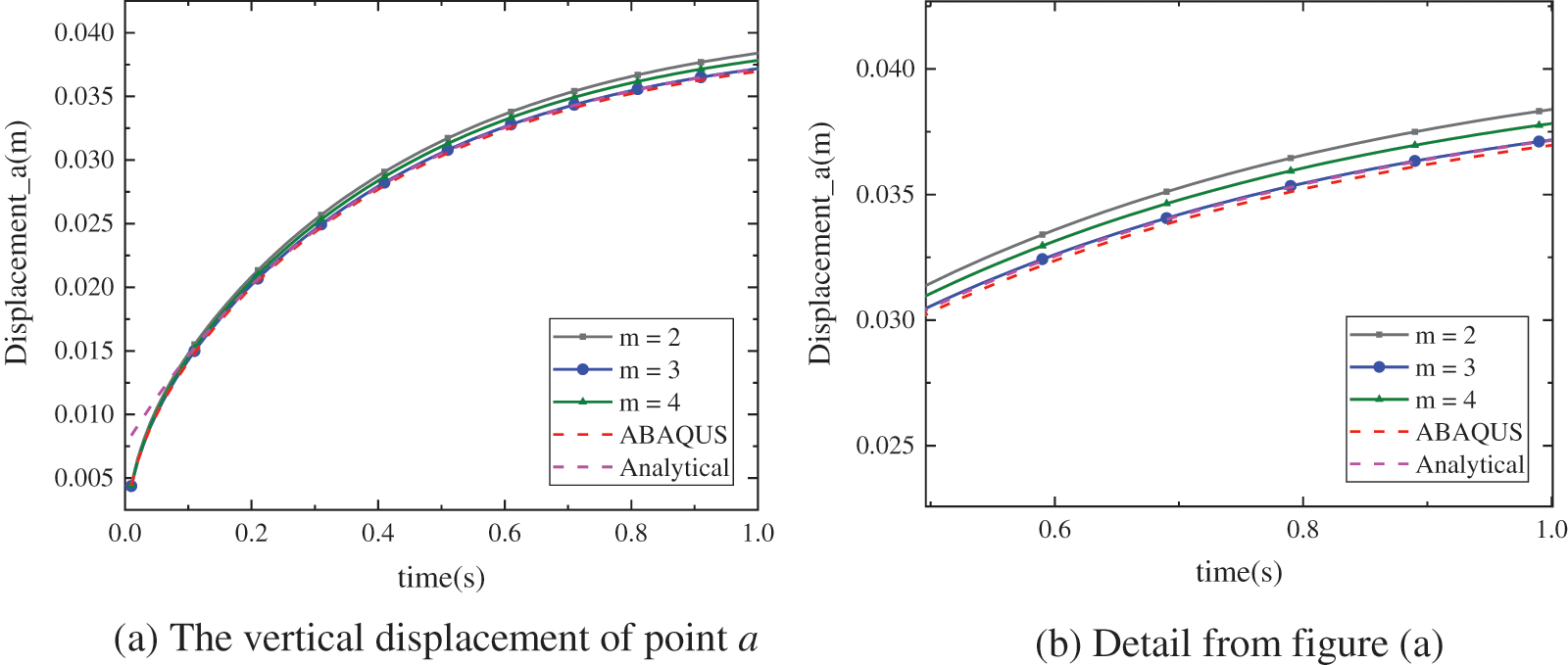Figure 7: The vertical displacement of point a, (b) is an enlarged detail from (a)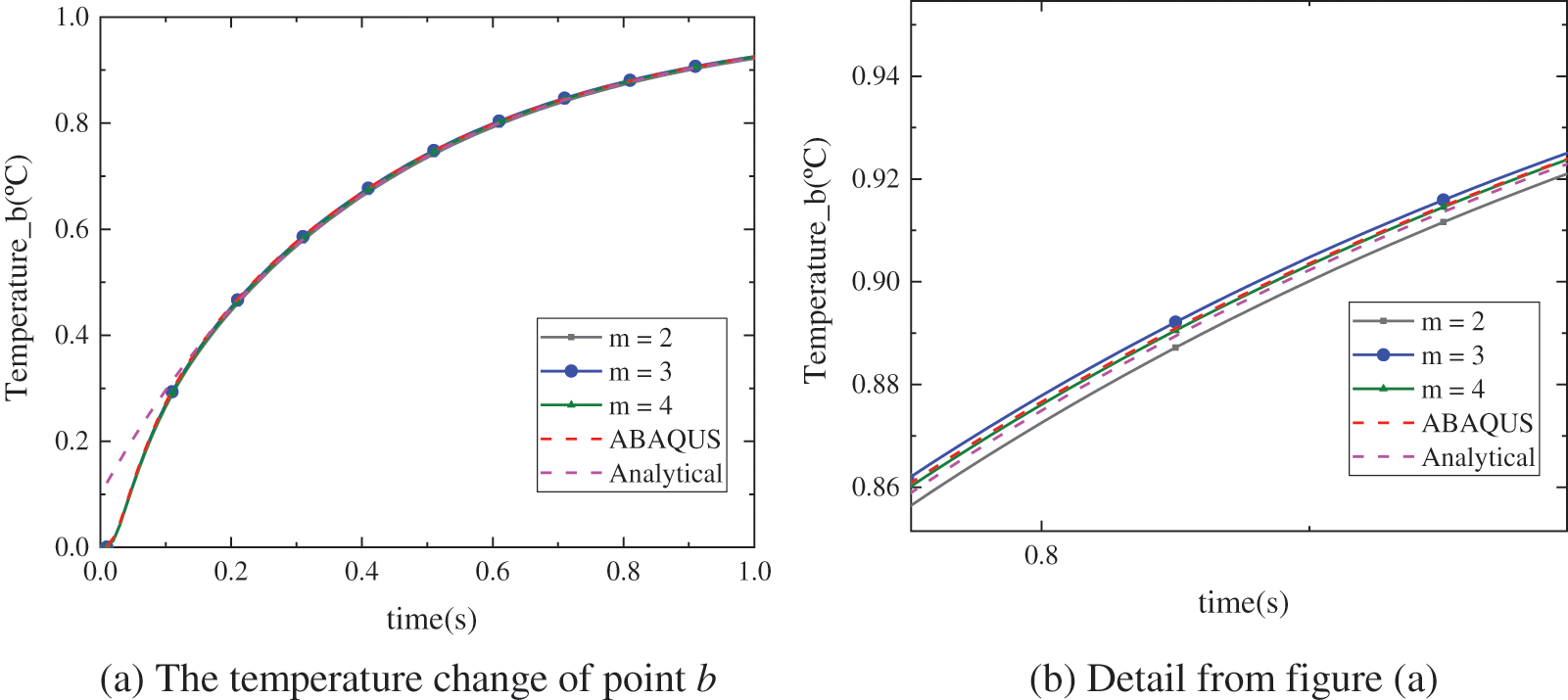Figure 8: The temperature change of point b, (b) is an enlarged detail from (a)

4.1.2 Influence of Particle Spacing

In addition to the influence of neighborhood range on the calculation results of bond-based peridynamic, the BB-PD model is also highly sensitive to particle spacing, i.e., the grid size. In this section, three different grid spacing will be selected in turn, i.e., Δx=0.005m, Δx=0.01m, Δx=0.02m, and the neighborhood size is taken as 3 times of particle spacing in Section 4.1.1, i.e., δ=3Δx. Figs. 9 and 10 show the displacement change diagram of point a in the vertical direction and the temperature change diagram of point b. It can be seen that when the neighborhood range is fixed as m = 3, the denser the grid distribution, the closer the displacement and temperature calculation results of PD are to the analytical solution and ABAQUS’s results.Figure 9: The vertical displacement of point a, (b) is an enlarged detail from (a)Figure 10: The temperature change of point b, (b) is an enlarged detail from (a)

In summary, when the horizon size is 3 times the particle spacing, the calculation results of the BB-PD model are accurate enough; when the particle spacing is smaller, the displacement and temperature calculation results are closer to the analytical solution and the calculation results of commercial software. The above results also prove that the bond-based peridynamic coupled thermo-chemo-mechanical numerical model given in this paper can be used to deal with the thermo-mechanical coupling response.

4.2 Verification of Damage Model

In this section, the crack growth process of the ceramic plate under cold shock is simulated and compared with the ceramic quenching experiment of Li et al.  to verify the BB-PD coupled thermo-chemo-mechanical model considering damage mentioned in this paper. In Li et al.’s experiment, the ceramic plate was heated to a certain temperature and rapidly dropped into the normal-temperature water. The high-temperature ceramic plate exhibited obvious shrinkage during quenching, forming several cracks on the surface. These parallel periodic cracks expand and drive inward. Referring to Li et al.’s experimental model , a quarter of the ceramic plate model is established. As shown in Fig. 11, the upper and right sides of the ceramic plate suffered cold shocks, and the displacement constraints in the x and y directions were applied on the left and lower sides of the ceramic plate, respectively.Figure 11: Geometry and boundary conditions of ceramic plates

The initial temperature of the ceramic plate is 773 K. The water temperature is 293 K and the coefficient of convection heat exchange is 70,000 W/(m2·K). Table 2 presents the material parameters of ceramic plates. In PD calculations, the distance between material points is Δ=0.05 mm, the time step is Δt=0.0001s and the neighborhood size is δ=3Δ.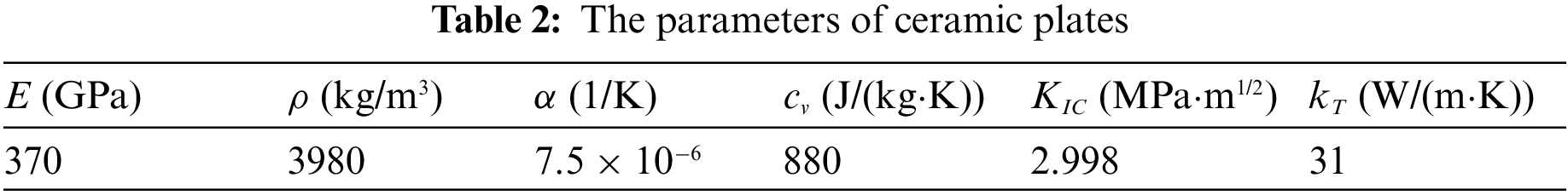Fig. 12 presents the crack path of the ceramic plate under cold shock. The comparison of Figs. 12a12c reveal that the PD-simulated crack path is basically same as that of Li et al.’s experimental  and numerical results , demonstrating the reliability of the BB-PD coupled thermo-chemo-mechanical model.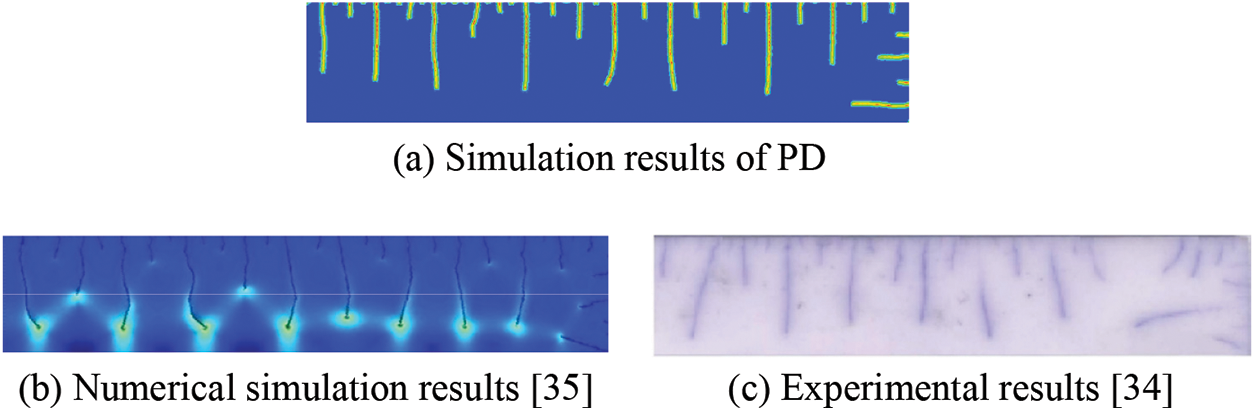Figure 12: The comparison of the final crack path

4.3 Verification of Oxidation Kinetics Model

In this section, the calculation model in Section 2.1 is used to simulate the ablation experiment of ZrB2 at different temperatures, and predict the growth and evolution of oxide layer thickness at different temperatures. The oxide layer thickness can be calculated by Eqs. (33) and (34). The proposed model results are compared with the experimental results of Opeka et al. . In addition, in the calculation of ZrB2 oxidation kinetics, the density of ZrO2 ceramics is taken as ρ=5600 kg/m3. The ambient oxygen concentration CO23 is set as 9.69 mol/m3.

When ZrB2 is oxidized in air for 5 h, the porosity (f) of oxide layer is 0.05 and the pore diameter (d) is 0.5 μm. Fig. 13 shows the relationship between oxidation temperature and oxide layer thickness. It can be found that the oxidation temperature and oxide layer thickness followed the parabolic trend. The effect of oxidation temperature on the oxide layer thickness is quite significant. When the oxidation temperature is 1273 K, the thickness of oxide layer is 14.6 μm, which increases to 75.3 and 321.0 μm at the oxidation temperature of 1473 and 1673 K, respectively. It can be seen that the growth of oxide layer thickness is obviously accelerated with the increase of oxidation temperature. In addition, it can be found that the predicted results of ZrB2 oxidation kinetics model are consistent with the experimental results, confirming that the proposed oxidation kinetics model can be used to simulate the growth in oxide thickness of ZrB2 ceramics at high temperatures.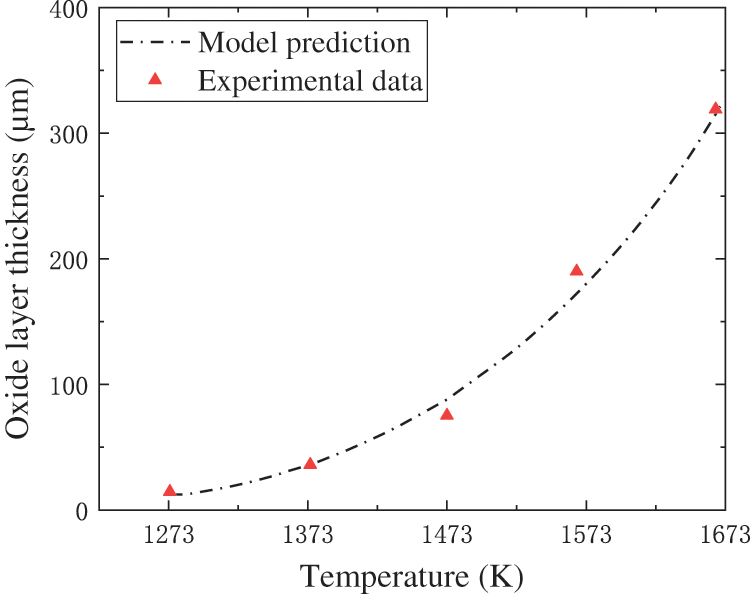Figure 13: The comparison between theoretically calculated and experimentally measured oxide layer thickness

4.4 Comparative Analysis of Coupled Thermo-Chemo-Mechanical and Coupled Thermo-Mechanical Calculations

In this section, the bond-based PD coupled thermo-chemo-mechanical model is used to simulate the dynamic response of ZrB2 ceramic plates under thermal shock. The boundary conditions of the ceramic plate are the same as those of Section 4.2. As shown in Fig. 14, the left and bottom boundaries are constrained in the x or y directions, and the top and right boundaries are subjected to thermal shock loads. The dimensions of ZrB2 ceramic plate are 0.015m×0.005m. The initial temperature is 293 K and the ambient temperature is 2293 K. The convection heat transfer coefficient is 70,000 W/(m2·K).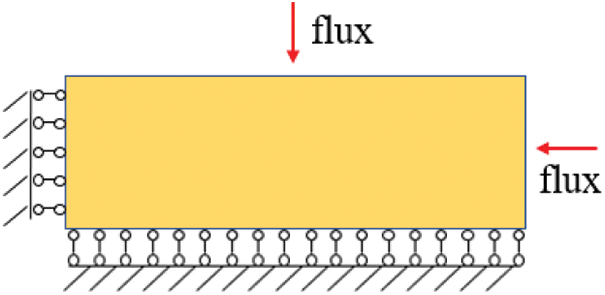Figure 14: The boundary conditions of ZrB2 ceramic plate

The distance between material points is Δ=0.00005m, the horizon size is δ=3Δ, and the time step is Δt=0.00001s. Table 3 presents the parameters of ZrB2 matrix and ZrO2 oxide layer. In order to analyze the influence of chemical reactions on the ablation process, the dynamic response of ZrB2 ceramic plate under fully coupled thermo-mechanical conditions is simulated.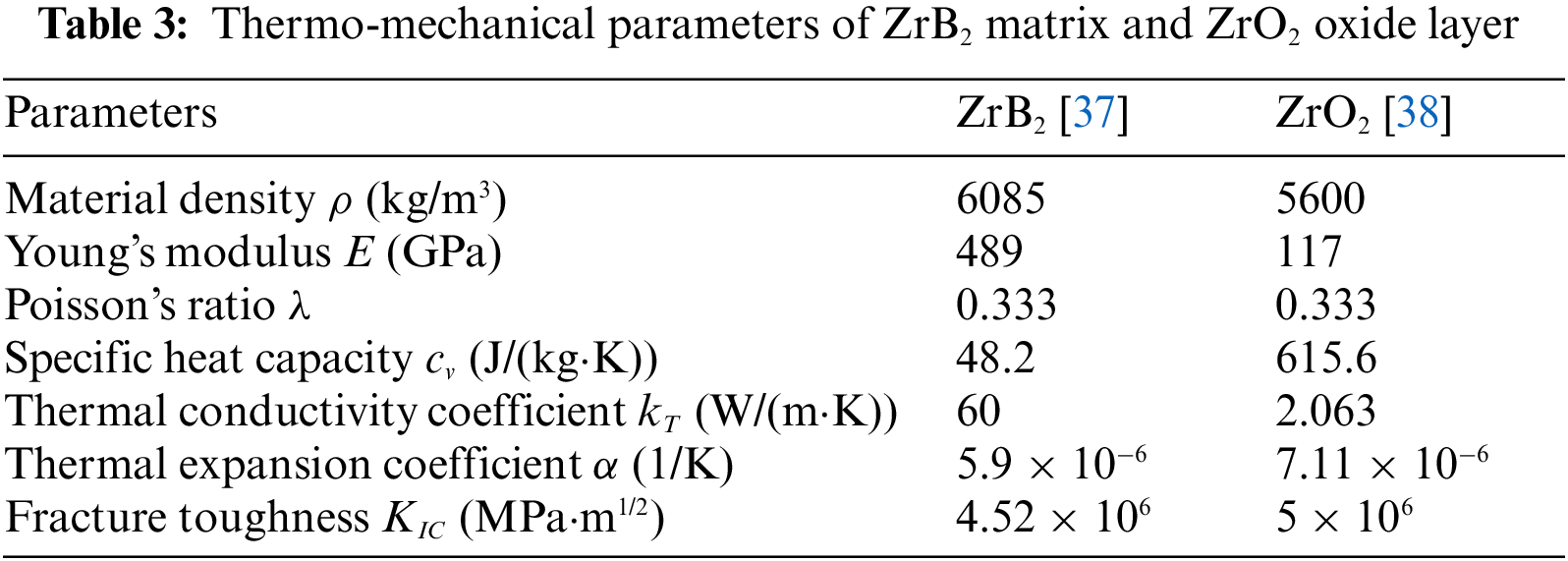Figs. 1517 present the temperature distribution, damage distribution diagrams of the ZrB2 ceramic plate, and compare with the temperature distribution results on the vertical centerline, obtained from both calculation methods. As shown in Fig. 15, the rate of temperature transfer of ZrB2 ceramic plate under thermo-chemo-mechanic coupling is lower than the thermo-mechanical coupling. According to Fig. 16, the maximum temperature of the ceramic plate with oxide layer is also lower than pure ZrB2 ceramic plate. It can be found that the ZrO2 oxide layer effectively resists the heat flow and inhibits temperature transfer on the ceramic plate to a certain extent, playing a certain protective role for the ZrB2 ceramic matrix. Fig. 17 shows the evolution of damage crack under two coupling calculations. When t = 2 ms, the model boundary damage and crack distribution are basically the same; When t = 4 ms, cracks begin to form in the ceramic plate without considering the evolution of oxide layer, but there is no crack initiation and development in the ceramic plate considering the evolution of oxide layer, which only shows that the local damage at the boundary develops to the inside; When t = 6 ms, the internal crack of the ceramic plate without considering the evolution of oxide layer continues to expand, while a crack appears in the upper left corner of the ceramic plate with considering the evolution of oxide layer, and the damage at the top boundary continues to expand inward; When t = 8 ms, the damage cracks of the two ceramic plates did not expand obviously. From the damage results of ceramic plates in Fig. 17, it can be concluded that the existence of oxide layer does enhance the thermal protection ability of ceramic plates and delay the damage caused by structural deformation caused by temperature change.Figure 15: The comparison of temperature (K) nephogram under (a) Thermo-mechanical coupling calculations and (b) Thermo-chemo-mechanical coupling calculationsFigure 16: The comparison of temperature distribution on the vertical centerline of ZrB2 ceramic plate under different calculation methods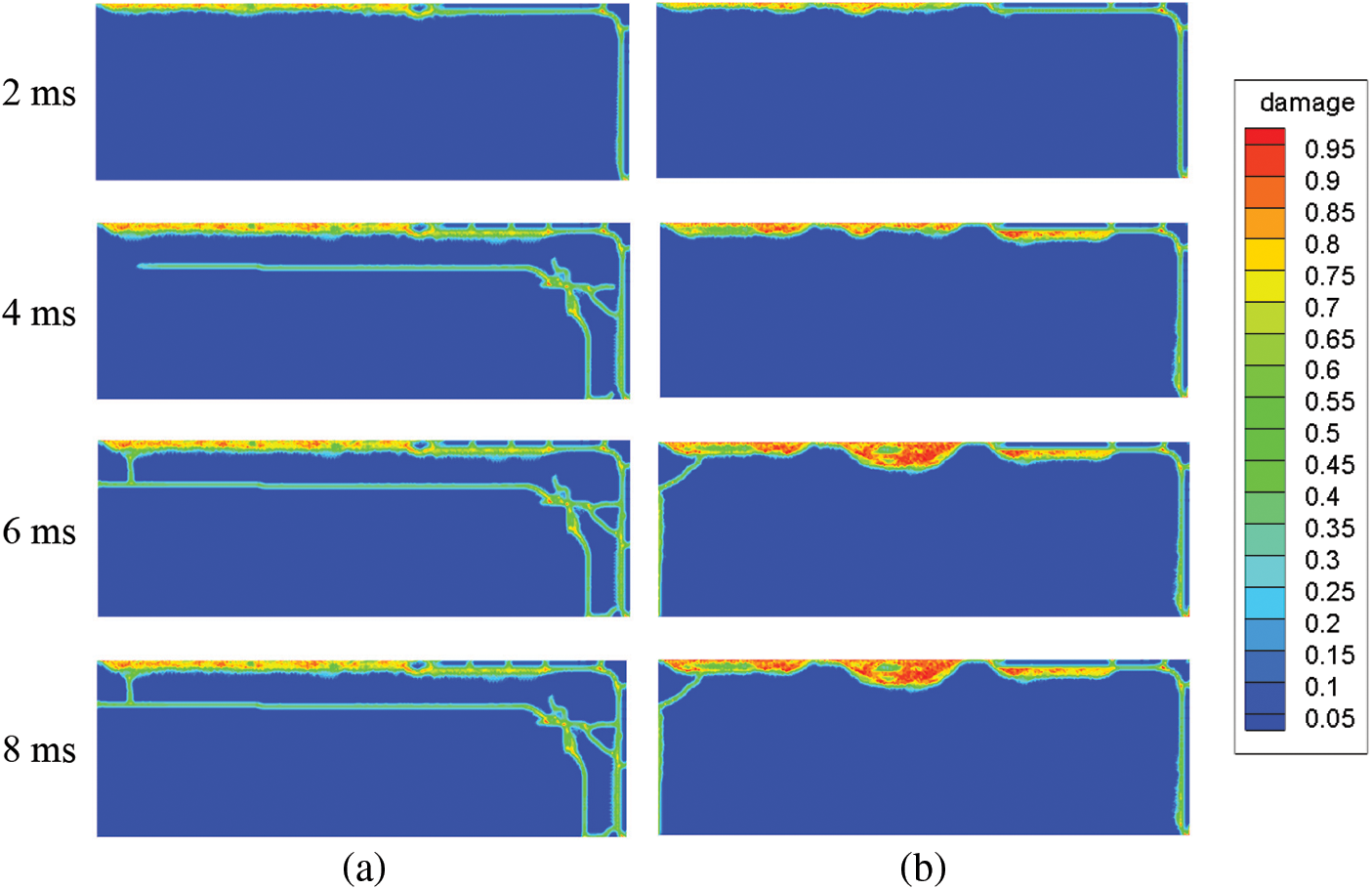Figure 17: The comparison of damage nephogram under (a) Thermo-mechanical coupling calculations and (b) Thermo-chemo-mechanical coupling calculations

From the above analysis, it can be seen that the evolution of oxide layer caused by chemical reaction during the ceramic ablation can indeed affect the thermal protection performance and damage development of ZrB2 ceramics, and the BB-PD coupled thermo-chemo-mechanical model based on ZrB2 ceramic ablation can deal with the problems of damage, fracture and temperature effect during the ZrB2 ceramic ablation to a certain extent.

5  Conclusions

Based on the bond-based peridynamic coupled thermo-mechanical theory and high-temperature oxidation kinetics model, a simple coupled thermo-chemo-mechanical model is established to simulate the ultrahigh-temperature ablation of ceramics. Compared with the coupled thermo-mechanical model, the coupled thermo-chemo-mechanical model considers the oxide layer generated by chemical reaction during ablation and the influence of chemical growth strain caused by the oxide layer on the bond basis force response function of PD. Finally, the influence of oxide layer on the ablation protection of ultra-high-temperature ceramics is analyzed in detail. The key results can be summarized as:

1.    The BB-PD coupled thermo-chemo-mechanical model based on the ablation of ultra-high-temperature ceramics is proposed, which can describe the heat conduction and damage process of ZrB2 ceramics under high-temperature ablation conditions. It is proved that this numerical model can be used to describe the high-temperature ablation process of ZrB2 ceramics, as verified by the cold impact damage experiment and evolution of oxidation layer thickness.

2.    The BB-PD coupled thermo-chemo-mechanical model is also used to simulate the high-temperature ablation process of ceramics. One should note that the results are significantly different from the calculation results of the BB-PD coupled thermo-mechanical, indicating that the presence of a protective oxide layer plays a key role in protecting the internal materials and preventing heat transfer.

In general, the bond-based peridynamic coupled thermo-chemo-mechanical model can describe the high-temperature ablation process of ZrB2 ceramics to a certain extent, revealing the changes in temperature distribution and damage behavior during the ceramic ablation.

Funding Statement: The authors acknowledge the support from the National Natural Science Foundation of China (11972267).

Conflicts of Interest: The authors declare that they have no conflicts of interest to report regarding the present study.

References

1.  1.  Zhang, X., Hu, P., Du, S., Han, J., Meng, S. (2015). Research progress on ultra-high temperature ceramic composites. Chinese Science Bulletin, 60(3), 257–266.
2.  2.  Kuriakose, A. K., Margrave, J. L. (1963). Kinetics of the reactions of elemental fluorine with zirconium carbide and zirconium diboride at high temperatures. Journal of Physical Chemistry, 68(2), 290–295.
3.  3.  Fahrenholtz, W. G., Hilmas, G. E., Talmy, I. G., Zaykoski, J. A. (2007). Refractory diborides of zirconium and hafnium. Journal of the American Ceramic Society, 90(5), 1347–1364.
4.  4.  Han, J., Hu, P., Zhang, X., Meng, S., Han, W. (2007). Oxidation-resistant ZrB2-SiC composites at 2200°C. Composites Science and Technology, 68(3), 799–806.
5.  5.  Wang, Y., Zhu, X., Zhang, L., Cheng, L. (2011). Reaction kinetics and ablation properties of C/C-ZrC composites fabricated by reactive melt infiltration. Ceramics International, 37(4), 1277–1283.
6.  6.  Qing, X., Sun, W., Tian, T., Xiong, X., Zhang, H. et al. (2020). Structural characteristics and ablative behavior of C/C-ZrC-SiC composites reinforced with Z-pins like Zr-Si-B-C multiphase ceramic rods. Ceramics International, 46(11), 18895–18902.
7.  7.  Fahrenholtz, W. G. (2005). The ZrB2 volatility diagram. Journal of the American Ceramic Society, 88(12), 3509–3512.
8.  8.  Talmy, I., Zaykoski, J., Opeka, M. (2008). Properties of ceramics in the ZrB2/ZrC/SiC system prepared by reactive processing. In: Ceramic engineering and science proceedings, vol. 19, no. 3, pp. 105–112. USA.
9.  9.  Fahrenholtz, W. G. (2007). Thermodynamic analysis of ZrB2-SiC oxidation: Formation of a SiC-depleted region. Journal of the American Ceramic Society, 90(1), 143–148.
10. 10. Parthasarathy, T., Rapp, R. A., Opeka, M., Kerans, R. J. (2008). A model for transitions in oxidation regimes of ZrB2. Materials Science Forum, 595(1), 823–832.
11. 11. Parthasarathy, T., Rapp, R., Opeka, M., Kerans, R. (2007). A model for the oxidation of ZrB2, HfB2 and TiB2. Acta Materialia, 55(17), 5999–6010.
12. 12. Zhou, Z., Peng, X., Wei, Z. (2014). A thermo-chemo-mechanical model for the oxidation of zirconium diboride. Journal of the American Ceramic Society, 98(2), 629–636.
13. 13. Wang, H., Shen, S. (2017). A chemomechanical coupling model for oxidation and stress evolution in ZrB2-SiC. Journal of Materials Research, 32(7), 1–12.
14. 14. Silling, S. (2000). Reformulation of elasticity theory for discontinuities and long-range forces. Journal of the Mechanics and Physics of Solids, 48(1), 175–209.
15. 15. Silling, S., Askari, E. (2005). A meshfree method based on the peridynamic model of solid mechanics. Computers and Structures, 83(17), 1526–1535.
16. 16. Oterkus, S., Madenci, E., Agwai, A. (2014). Fully coupled peridynamic thermomechanics. Journal of the Mechanics and Physics of Solids, 64(1), 1–23.
17. 17. Liao, Y., Liu, L., Liu, Q., Lai, X., Assefa, M. et al. (2017). Peridynamic simulation of transient heat conduction problems in functionally gradient materials with cracks. Journal of Thermal Stresses, 40(12), 1–18. DOI 10.1080/01495739.2017.1358070.
18. 18. Wang, Y., Zhou, X. (2019). Peridynamic simulation of thermal failure behaviors in rocks subjected to heating from boreholes. International Journal of Rock Mechanics and Mining Sciences, 117(1–2), 31–48. DOI 10.1016/j.ijrmms.2019.03.007.
19. 19. Wang, Y., Zhou, X., Zhang, T. (2019). Size effect of thermal shock crack patterns in ceramics: Insights from a nonlocal numerical approach. Mechanics of Materials, 137(8), 103133. DOI 10.1016/j.mechmat.2019.103133.
20. 20. Chen, W., Gu, X., Zhang, Q., Xia, X. (2021). A refined thermo-mechanical fully coupled peridynamics with application to concrete cracking. Engineering Fracture Mechanics, 242(8), 107463. DOI 10.1016/j.engfracmech.2020.107463.
21. 21. Cheng, Z., Wang, Z., Luo, Z. (2019). Dynamic fracture analysis for shale material by peridynamic modelling. Computer Modeling in Engineering & Sciences, 118(3), 509–527. DOI 10.31614/cmes.2019.04339.
22. 22. Chu, B., Liu, Q., Liu, L., Lai, X., Mei, H. (2020). A rate-dependent peridynamic model for the dynamic behavior of ceramic materials. Computer Modeling in Engineering & Sciences, 124(1), 151–178. DOI 10.32604/cmes.2020.010115.
23. 23. Wang, Y., Zhou, X., Kou, M. (2019). An improved coupled thermo-mechanic bond-based peridynamic model for cracking behaviors in brittle solids subjected to thermal shocks. European Journal of Mechanics-A/solids, 73(3), 282–305. DOI 10.1016/j.euromechsol.2018.09.007.
24. 24. Wang, Y., Zhou, X., Kou, M. (2018). Peridynamic investigation on thermal fracturing behavior of ceramic nuclear fuel pellets under power cycles. Ceramics International, 44(10), 11512–11542. DOI 10.1016/j.ceramint.2018.03.214.
25. 25. Yu, H., Li, S. (2020). On energy release rates in peridynamics. Journal of the Mechanics and Physics of Solids, 142(3), 104024. DOI 10.1016/j.jmps.2020.104024.
26. 26. Song, Y., Li, S., Zhang, S. (2021). Peridynamic modeling and simulation of thermo-mechanical de-icing process with modified ice failure criterion. Defence Technology, 17(1), 15–35. DOI 10.1016/j.dt.2020.04.001.
27. 27. Li, J., Li, S., Lai, X., Liu, L. (2022). Peridynamic stress is the static first piola-kirchhoff virial stress. International Journal of Solids and Structures, 241(1–2), 111478. DOI 10.1016/j.ijsolstr.2022.111478.
28. 28. Loeffel, K., Anand, L. (2011). A chemo-thermo-mechanically coupled theory for elastic-viscoplastic deformation, diffusion, and volumetric swelling due to a chemical reaction. International Journal of Plasticity, 27(9), 1409–1431. DOI 10.1016/j.ijplas.2011.04.001.
29. 29. Xu, G., Yang, L., Zhou, Y., Pi, Z., Zhu, W. (2019). A chemo-thermo-mechanically constitutive theory for thermal barrier coatings under CMAS infiltration and corrosion. Journal of the Mechanics and Physics of Solids, 133(1), 1–19. DOI 10.1016/j.jmps.2019.103710.
30. 30. Clarke, D. (2003). The lateral growth strain accompanying the formation of a thermally grown oxide. Acta Materialia, 51(5), 1393–1408. DOI 10.1016/S1359-6454(02)00532-3.
31. 31. Lee, J., Oh, S. E., Hong, J. (2017). Parallel programming of a peridynamics code coupled with finite element method. International Journal of Fracture, 203(1), 99–114. DOI 10.1007/s10704-016-0121-y.
32. 32. Timoshenko, S. P., Goodier, J. N. (1970). Theory of elasticity. New York: Mcgraw-Hill.
33. 33. Carslaw, H. S., Jaeger, J. C. (1959). Conduction of heat in solids. Oxford: Clarendon Press.
34. 34. Li, J., Song, F., Jiang, C. (2013). Direct numerical simulations on crack formation in ceramic materials under. Journal of the European Ceramic Society, 33(13), 2677–2687. DOI 10.1016/j.jeurceramsoc.2013.04.012.
35. 35. Li, J., Song, F., Jiang, C. (2015). A non-local approach to crack process modeling in ceramic materials subjected to thermal shock. Engineering Fracture Mechanics, 133(4), 85–98. DOI 10.1016/j.engfracmech.2014.11.007.
36. 36. Opeka, M. M., Talmy, I. G., Wuchina, E. J., Zaykoski, J. A., Causey, S. J. (1999). Mechanical, thermal, and oxidation properties of refractory hafnium and zirconium compounds. Journal of the European Ceramic Society, 19(13), 2405–2414. DOI 10.1016/S0955-2219(99)00129-6.
37. 37. Justin, J. F., Jankowiak, A. (2011). Ultra high temperature ceramics: Densification, properties and thermal stability. High Temperature Materials, 1(3), 1–12.
38. 38. Tan, Y. (2020). Peridynamic simulation of functionally graded materials under thermal shock loading (Master Thesis). Wuhan, China: Wuhan University of Technology.

Li, Y., Liu, Q., Liu, L., Mei, H. (2023). The Coupled Thermo-Chemo-Mechanical Peridynamics for ZrB2 Ceramics Ablation Behavior. CMES-Computer Modeling in Engineering & Sciences, 135(1), 417–439.This work is licensed under a Creative Commons Attribution 4.0 International License , which permits unrestricted use, distribution, and reproduction in any medium, provided the original work is properly cited.

View

• ### 0

Like

Related articles
• LUZMARINA HERNANDES*, LUCIENI...
• Liang Yang, Caizhuang Wang, Shiwei...
• Taotao Hu, gping Shen
• T.I. Zohdi
• Leonid V. Zhigilei, Avinash M....